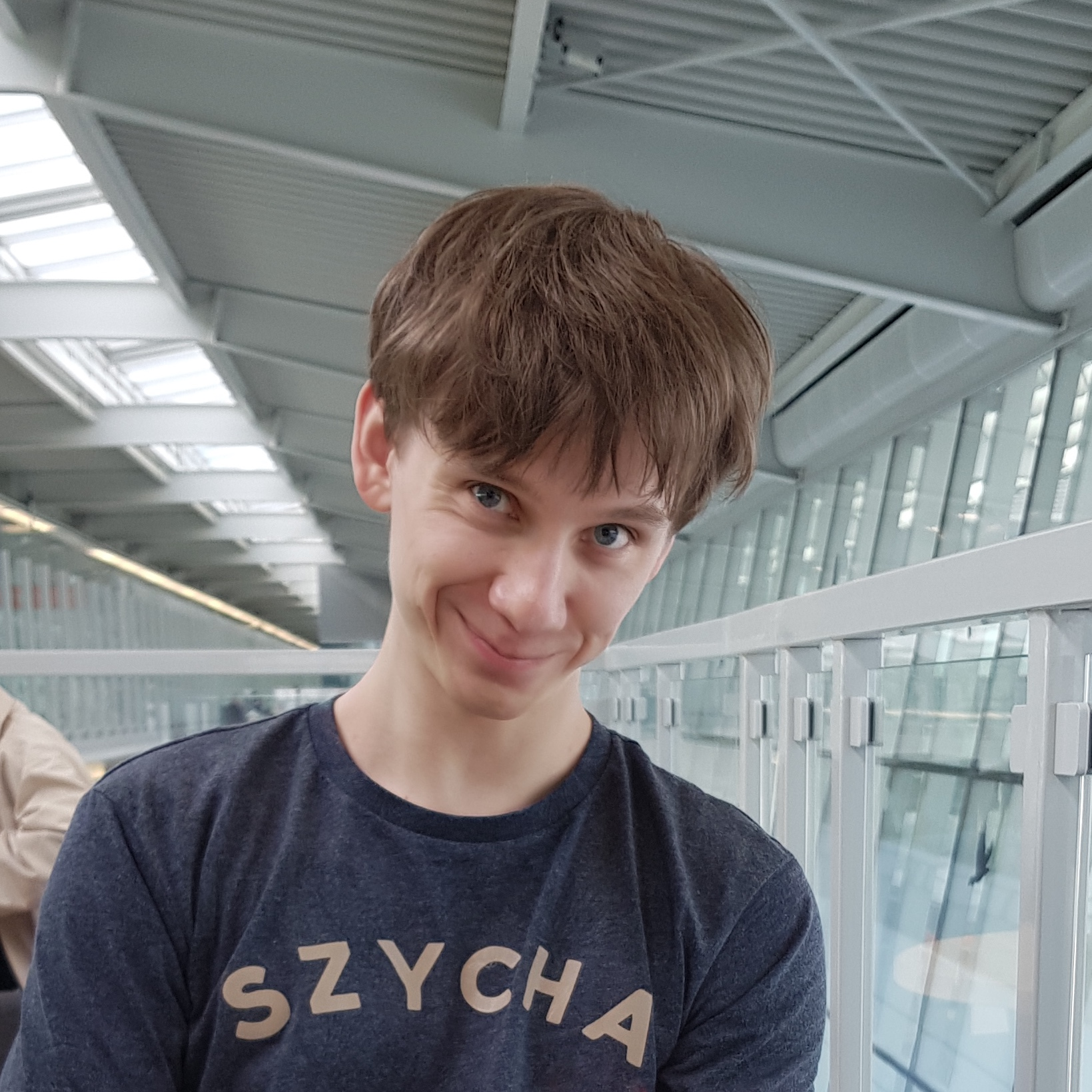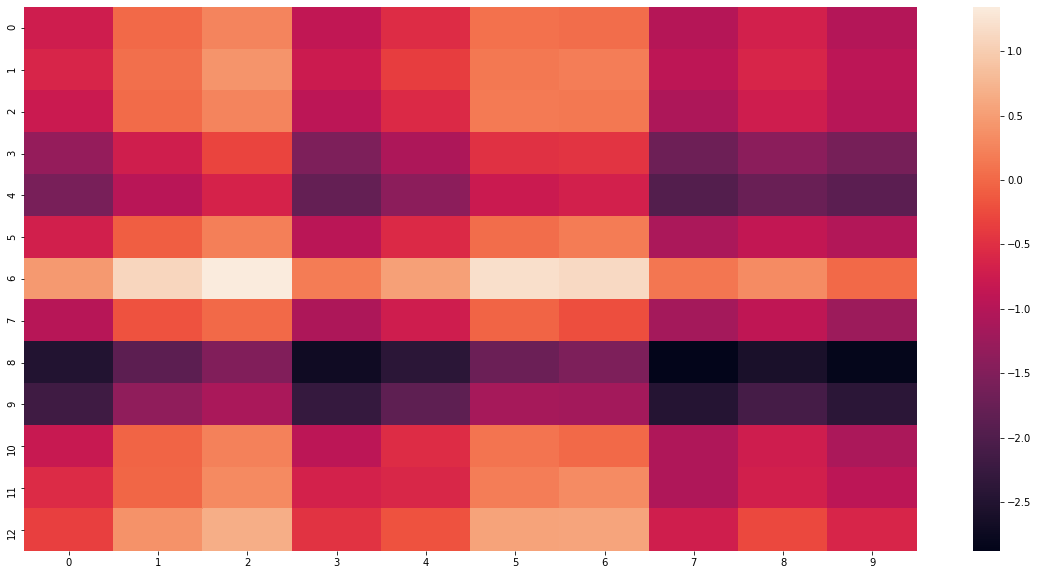### Tomek Korbak

PhD student,
University of Sussex

# Implementing additive and multiplicative attention in PyTorch

Attention mechanisms revolutionized machine learning in applications ranging from NLP through computer vision to reinforcement learning. Attention is the key innovation behind the recent success of Transformer-based language models1 such as BERT.2 In this blog post, I will look at a two initial instances of attention that sparked the revolution — additive attention (also known as Bahdanau attention) proposed by Bahdanau et al3 and multiplicative attetion (also known as Luong attention) proposed by Luong et al.4

The idea of attention is quite simple: it boils down to weighted averaging. Let us consider machine translation as an example. When generating a translation of a source text, we first pass the source text through an encoder (an LSTM or an equivalent model) to obtain a sequence of encoder hidden states $$\mathbf{s}_1, \dots, \mathbf{s}_n$$. Then, at each step of generating a translation (decoding), we selectively attend to these encoder hidden states, that is, we construct a context vector $$\mathbf{c}_i$$ that is a weighted average of encoder hidden states:

$\mathbf{c}_i = \sum\limits_j a_{ij}\mathbf{s}_j$

We choose the weights $$a_{ij}$$ based both on encoder hidden states $$\mathbf{s}_1, \dots, \mathbf{s}_n$$ and decoder hidden states $$\mathbf{h}_1, \dots, \mathbf{h}_m$$ and normalize them so that they encode a categorical probability distribution $$p(\mathbf{s}_j \vert \mathbf{h}_i)$$.

$\mathbf{a}_i = \text{softmax}(f_\text{att}(\mathbf{h}_i, \mathbf{s}_j))$

Intuitively, this corresponds to assigning each word of a source sentence (encoded as $$\mathbf{s}_j$$) a weight $$a_{ij}$$ that tells how much the word encoded by $$\mathbf{s}_j$$ is relevant for generating subsequent $$i$$th word (based on $$\mathbf{h}_i$$) of a translation. The weighting function $$f_\text{att}(\mathbf{h}_i, \mathbf{s}_j)$$ (also known as alignment function or score function) is responsible for this credit assignment. The PyTorch snippet below provides an abstract base class for attention mechanism.

class Attention(torch.nn.Module):

def __init__(self, encoder_dim: int, decoder_dim: int):
super().__init__()
self.encoder_dim = encoder_dim
self.decoder_dim = decoder_dim

def forward(self,
query: torch.Tensor,  # [decoder_dim]
values: torch.Tensor, # [seq_length, encoder_dim]
):
weights = self._get_weights(query, values) # [seq_length]
weights = torch.nn.functional.softmax(weights, dim=0)
return weights @ values  # [encoder_dim]


Here _get_weights corresponds to $$f_\text{att}$$, query is a decoder hidden state $$\mathbf{h}_i$$ and values is a matrix of encoder hidden states $$\mathbf{s}$$. To keep the illustration clean, I ignore the batch dimension.

There are many possible implementations of $$f_\text{att}$$ (_get_weights). In this blog post, I focus on two simple ones: additive attention and multiplicative attention.

Additive attention uses a single-layer feedforward neural network with hyperbolic tangent nonlinearity to compute the weights $$a_{ij}$$:

$f_\text{att}(\mathbf{h}_i, \mathbf{s}_j) = \mathbf{v}_a{}^\top \text{tanh}(\mathbf{W}_1 \mathbf{h}_i + \mathbf{W}_2 \mathbf{s}_j),$

where $$\mathbf{W}_1$$ and $$\mathbf{W}_2$$ are matrices corresponding to the linear layer and $$\mathbf{v}_a$$ is a scaling factor. In PyTorch snippet below I present a vectorized implementation computing attention mask for the entire sequence $$\mathbf{s}$$ at once.

class AdditiveAttention(Attention):

def __init__(self, encoder_dim, decoder_dim):
super().__init__(encoder_dim, decoder_dim)
self.v = torch.nn.Parameter(
torch.FloatTensor(self.decoder_dim).uniform_(-0.1, 0.1))
self.W_1 = torch.nn.Linear(self.decoder_dim, self.decoder_dim)
self.W_2 = torch.nn.Linear(self.encoder_dim, self.decoder_dim)

def _get_weights(self,
query: torch.Tensor,  # [decoder_dim]
values: torch.Tensor,  # [seq_length, encoder_dim]
):
query = query.repeat(values.size(0), 1)  # [seq_length, decoder_dim]
weights = self.W_1(query) + self.W_2(values)  # [seq_length, decoder_dim]


## Multiplicative attention

Multiplicative attention is the following function:

$f_\text{att}(\mathbf{h}_i, \mathbf{s}_j) = \mathbf{h}_i{}^\top \mathbf{W} \mathbf{s}_j,$

where $$\mathbf{W}$$ is a matrix. It essentially encodes a bilinear form of the query and the values and allows for multiplicative interaction of query with the values, hence the name. Additionally, Vaswani et al.1 advise to scale the attention scores by the inverse square root of the dimensionality of the queries.

Again, a vectorized implementation computing attention mask for the entire sequence $$\mathbf{s}$$ is below.

class MultiplicativeAttention(Attention):

def __init__(self, encoder_dim: int, decoder_dim: int):
super().__init__(encoder_dim, decoder_dim)
self.W = torch.nn.Parameter(torch.FloatTensor(
self.decoder_dim, self.encoder_dim).uniform_(-0.1, 0.1))

def _get_weights(self,
query: torch.Tensor,  # [decoder_dim]
values: torch.Tensor, # [seq_length, encoder_dim]
):
weights = query @ self.W @ values.T  # [seq_length]
return weights/np.sqrt(self.decoder_dim)  # [seq_length]


## Using attention

In practice, the attention mechanism handles queries at each time step of text generation.

attention = MultiplicativeAttention(encoder_dim=100, decoder_dim=50)
decoder = torch.nn.LSTMCell(100, 50)
encoder_hidden_states = torch.rand(10, 100)
h, c = torch.rand(1, 50), torch.rand(1, 50)
for step in range(13):
context_vector = attention(h.squeeze(0), encoder_hidden_states)
(h, c) = decoder(context_vector.unsqueeze(0), (h, c))
# Generating the next work based on h


Here context_vector corresponds to $$\mathbf{c}_i$$. h and c are LSTM’s hidden states, not crucial for our present purposes.

Finally, it is now trivial to access the attention weights $$a_{ij}$$ and plot a nice heatmap.

encoder_dim, decoder_dim, encoder_seq_length, decoder_seq_length = 100, 50, 10, 13
encoder_hidden_states = torch.rand(encoder_seq_length, encoder_dim)
decoder_hidden_states = torch.rand(decoder_seq_length, decoder_dim)
weights = torch.FloatTensor(decoder_seq_length, encoder_seq_length)
for step in range(decoder_seq_length):
context_vector = attention(decoder_hidden_states[step], encoder_hidden_states)
weights[step] = attention._get_weights(decoder_hidden_states[step], encoder_hidden_states)
seaborn.heatmap(weights.detach().numpy())Here each cell corresponds to a particular attention weight $$a_{ij}$$. For a trained model and meaningful inputs, we could observe patterns there, such as those reported by Bahdanau et al.3 — the model learning the order of compound nouns (nouns paired with adjectives) in English and French. Let me end with this illustration of the capabilities of additive attention.